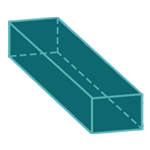Report a Problem
Suggestions

#Calculate The Surface Area of A Cuboid

Last updated: Saturday, June 24, 2023

The surface area of a cuboid is the sum of the areas of all of its faces. A cuboid has six faces, each of which is a rectangle. To find the surface area, you need to find the area of each rectangle and then add them together.

Real-life examples of cuboids include shoeboxes, books, and refrigerators. When designing packaging for products, manufacturers need to know the surface area of the cuboid packaging in order to determine the amount of material needed to cover it. Architects and builders also need to calculate the surface area of cuboid-shaped rooms and buildings to determine how much paint, wallpaper, or siding is needed to cover the surfaces.

The formula for determining the surface area of a cuboid is defined as:
$$SA$$ $$=$$ $$2$$ $$\cdot$$ $$L$$ $$\cdot$$ $$W$$ $$+$$ $$2$$ $$\cdot$$ $$L$$ $$\cdot$$ $$D$$ $$+$$ $$2$$ $$\cdot$$ $$W$$ $$\cdot$$ $$D$$
$$SA$$: the surface area of the cuboid
$$L$$: the length of the longer side
$$W$$: the length of the shorter side
$$D$$: the length of the depth
The SI unit of surface area is: $$square \text{ } meter\text{ }(m^2)$$

## Find $$SA$$

Use this calculator to determine the surface area of a cuboid when the length of all sides are given.
Hold & Drag
CLOSE
the length of the longer side
$$L$$
$$meter$$
the length of the shorter side
$$W$$
$$meter$$
the length of the depth
$$D$$
$$meter$$
Bookmark this page or risk going on a digital treasure hunt again